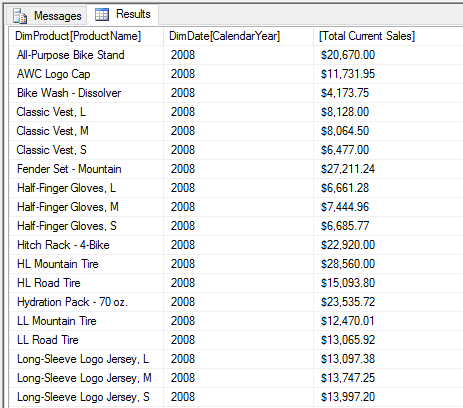# SSAS Calculated Measures

## SSAS - Calculated Measures and Usage in MDX and DAX Queries

This article describes how to create calculated measures in an SSAS Cube for use in MDX queries and in an SSAS Model of a Tabular Database for use in DAX queries. Upon adding each Calculated Measure in the Cube and Model Visual Studio projects, these projects will be Deployed and Processed to update the database and then in SSMS, the database will need to be Refreshed to see the newly added Calculated Measures.

## Creating a Calculated Measure in a Cube

To create calculated measures in a Cube, double click the Calculations tab in the Cube in Visual Studio.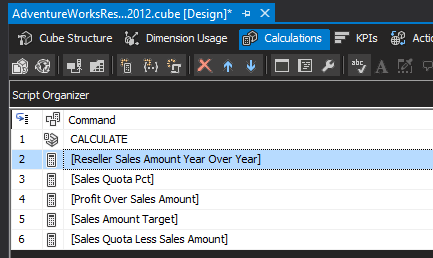Right click on the Calculate item and select New Calculated Member to get a blank Form View or click the New Calculated Member button (Highlighted in Yellow).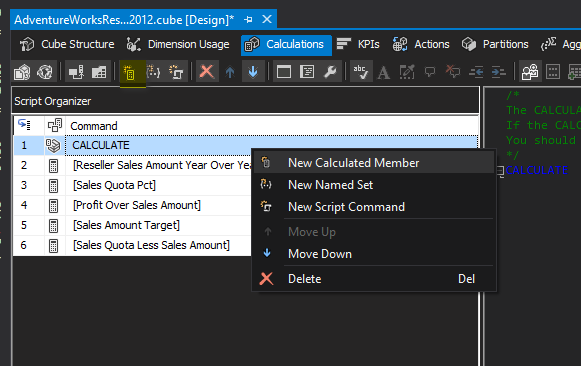Enter a Name for the Calculated measure and Expression. You can add the Format string and Associated Measure Group as well as Visible property and Non-empty behavior here as well. This measure is a calculation to display profit remaining after the Sales Amount has the Total Product Cost subtracted from it.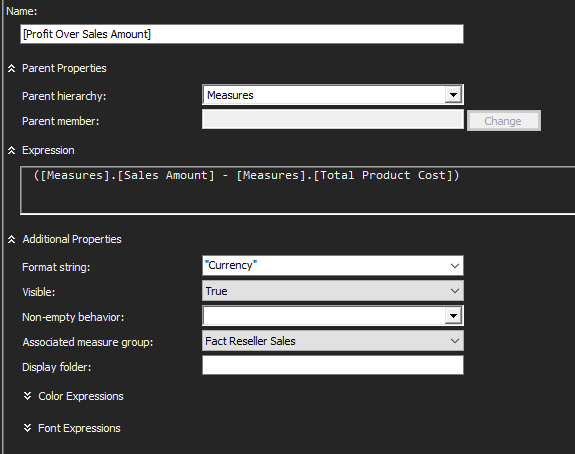## Writing an MDX Query with a Calculated Measures

After Saving the cube and Deploying and Processing it, open SSMS in Analysis Services to see the new Measure. The MDX Query below uses the newly created measure and Sales Amount from the Fact Reseller Sales measure as Columns and extracts the Category Name as the Row. Note that this query does not specify Members, Children, CurrentMember, etc. so that the row reads for All categories.

select {
[Measures].[Profit Over Sales Amount],
[Measures].[Sales Amount]} on 0,
[Dim Product Category].[Category Name] on 1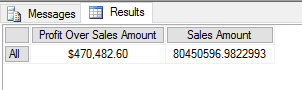By adding .Members to the Category Name, the individual categories appear in the rows in the Query.

select {
[Measures].[Profit Over Sales Amount],
[Measures].[Sales Amount]} on 0,
[Dim Product Category].[Category Name].Members on 1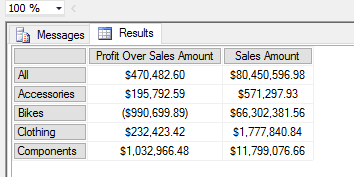This next Calculated Measure uses the Sales Quota Amount measure and subtracts the Sales Amount from it to get the difference.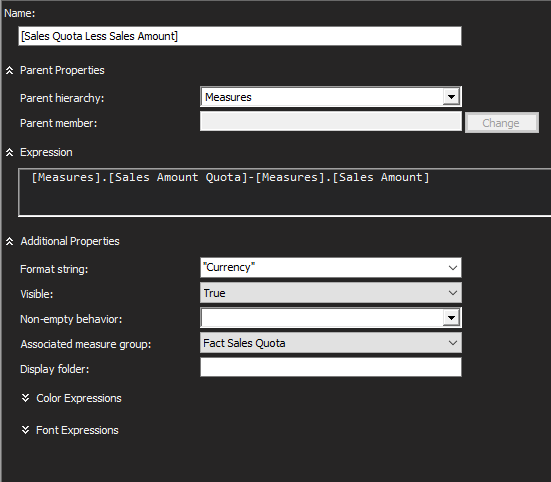In an MDX query, we can show the Sales Amount Quota for each Employee as well as their Sales Amounts and the calculated measure showing the difference.

select { [Measures].[Fact Sales Quota Count],
[Measures].[Sales Amount Quota],
[Measures].[Sales Amount],
[Measures].[Sales Quota Less Sales Amount]} on columns,
{ [Dim Employee].[Full Name].Members
} on rows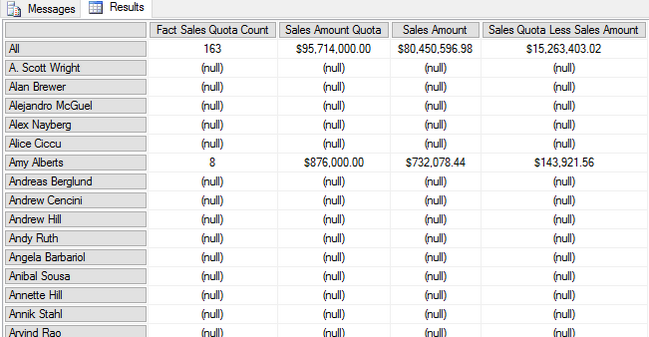If the amounts for Sales Quota and Sales Amount did not have their Format String property set to Currency, we could rewrite the query to add a couple of Members with formatted Strings for each one. Also added was the non empty clause to the Employees to filter out only those employees who have Sales Quotas and Sales Amounts.

with
member [Measures].[Sales Quota] as [Measures].[Sales Amount Quota], Format_String="Currency"
member [Measures].[Sales Total] as [Measures].[Sales Amount], Format_String="Currency"

select { [Measures].[Fact Sales Quota Count],
[Measures].[Sales Quota],
[Measures].[Sales Total],
[Measures].[Sales Quota Less Sales Amount]} on columns,
non empty{ [Dim Employee].[Full Name].Members
} on rows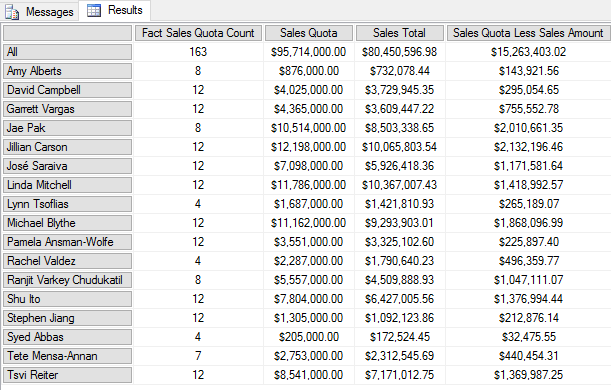Another Calculated Measure to compute the Percentage of the Sales Amount to the Sales Amount Quota target is added here.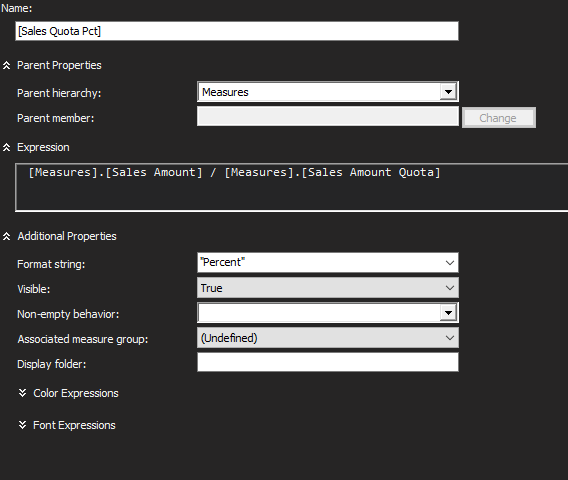The MDX query is using a cross join on the Calendar Year and Sales Quota Percent to determine what percentage of the Sales Quota for each Employee was met.

select
[Order Date].[Calendar Year].children * [Measures].[Sales Quota Pct] on columns,
nonempty ( [Dim Employee].[Full Name].children ) on rows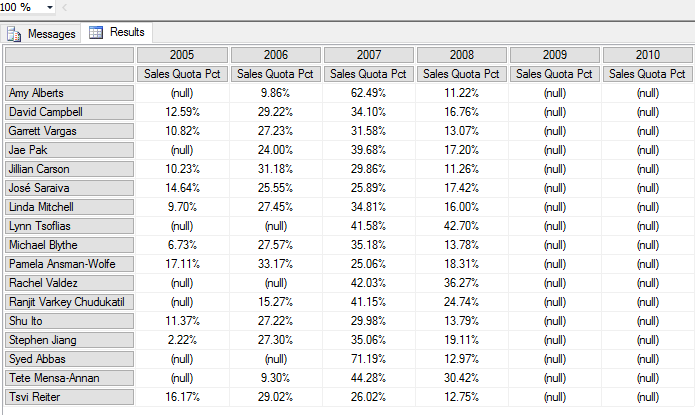The next calculated measure will be for a Reseller Sales Amount that takes the Current Year provided amount and subtracts the previous year Sales amount to get the difference of Sales amounts Year over Year. The calculation will display either a Profit or Loss between the two years.

First, we will get the Reseller Sales Amounts

select {[Measures].[Sales Amount]} on 0,
{[Order Date].[Calendar Year].children} on 1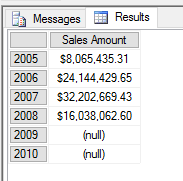The calculated measure will use an expression that includes the Order Date dimension Calendar Year and Sales Amount: one for the currentMember subtracted by the PreviousMember.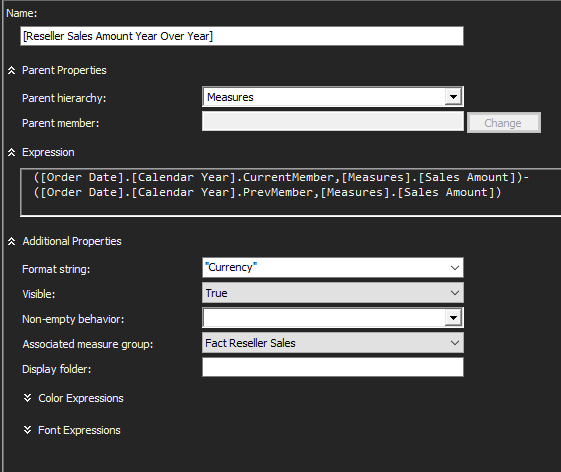The MDX query will simply use the Calculated Measure for the Reseller Sales Amounts with a Where clause with the CurrentMember Year provided. In this case, the year 2006.

select
[Measures].[Reseller Sales Amount Year Over Year] on columns
where [Order Date].[Calendar Year].&;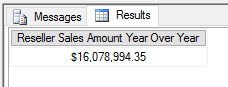The results provided by the query take the Sales Amount of 2006 subtracted by the Sales Amount of 2005. In the snapshot from Excel below, we can see that the amount equals the output from the query.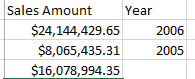By changing the Calendar Year in the Where clause, the CurrentMember is changed to 2007 and the PreviousMember to 2006 for this calculation.

select
[Measures].[Reseller Sales Amount Year Over Year] on columns
where [Order Date].[Calendar Year].&;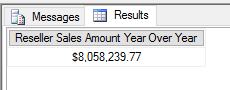## DAX Queries and Tabular Database Model Measures

Setting up Calculated Measures is quite easy. By clicking on Table in the Menu bar and clicking on Show Measure Grid, the area below each tab in the project will appear. This is where the measures are added.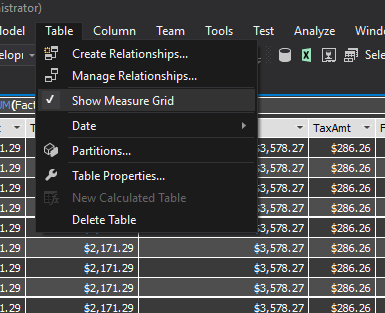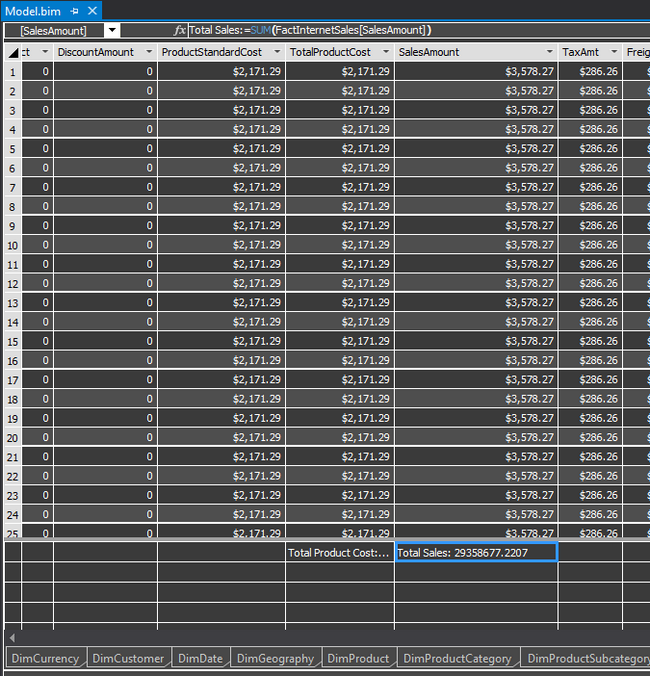The 2 measures that were added that will be used in a DAX query are Total Sales (located at the bottom of the SalesAmount Column) and Total Units (located at the bottom of the OrderQuantity column)

Syntax:
Total Sales:=SUM(FactInternetSales[SalesAmount])
Total Units:=SUM(FactInternetSales[OrderQuantity])

--using Total Sales, Total Units on FactInternetSales

evaluate
(
summarize
(
'FactInternetSales',
DimProductCategory[CategoryName],
DimProduct[ProductName],
DimDate[CalendarYear],
"Total Sales", format(FactInternetSales[Total Sales], "Currency"),
"Total Units", FactInternetSales[Total Units]
)
)
order by DimProduct[ProductName],DimDate[CalendarYear]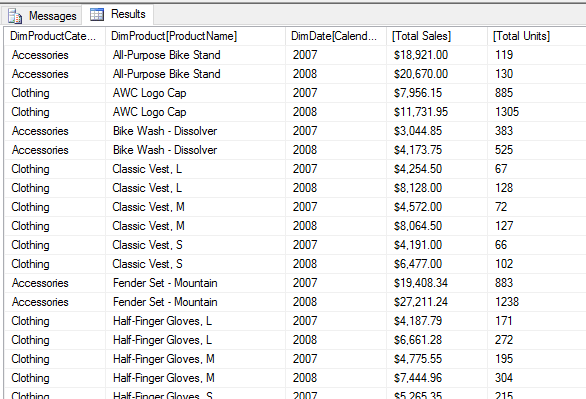Internet Current Total Sales Measure: On the left side of the Measures Grid, click in a cell and type:
Internet Curr Total Sales:=TOTALYTD([Total Sales],[DimDate[FullDate])
The information highlighted in the Model View shows how it appears in Visual Studio.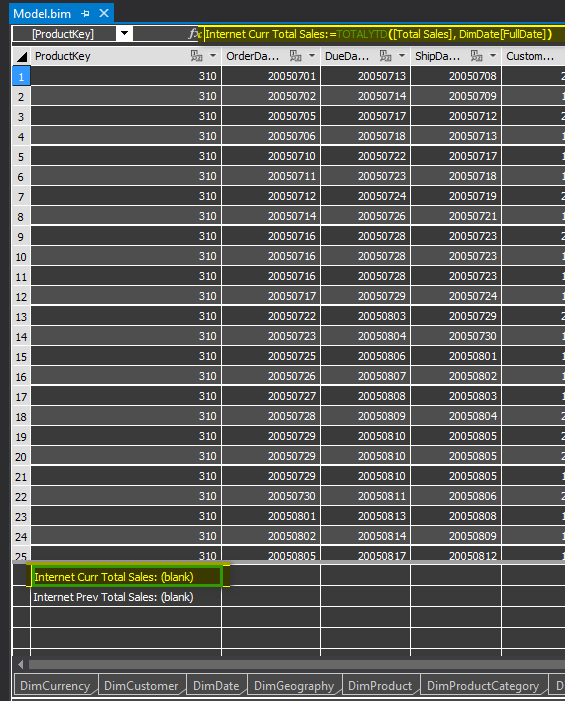The DAX Query that uses this calculated measure will display the Product Name, Calendar Year, and the Total Current Sales for that product in the calendar year provided. By using a SUMX function with a FILTER function, the Calendar Year may be applied to the query.

--Internet Curr Total Sales Calc Measure
evaluate
(
summarize
(
'FactInternetSales',
DimProduct[ProductName],
DimDate[CalendarYear],
"Total Current Sales", format(sumx(Filter('FactInternetSales',DimDate[CalendarYear]=2008), FactInternetSales[Internet Curr Total Sales]), "Currency")
)
)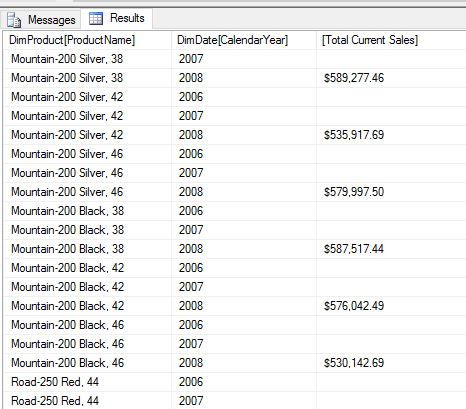Only the rows with the matching Calendar Year display data. To remove the rows without data, a filter may be applied to show only the DimDate CalendarYear of 2008 as shown below.

evaluate
(
filter
(
summarize
(
'FactInternetSales',
DimProduct[ProductName],
DimDate[CalendarYear],
"Total Current Sales", format(sumx(Filter('FactInternetSales',DimDate[CalendarYear]=2008),
FactInternetSales[Internet Curr Total Sales]), "Currency")
), DimDate[CalendarYear]=2008
)
)order by DimProduct[ProductName];

This result set displays only products with sales amounts for the year 2008 only.